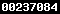### The IMPLEMENTATION of LEARNING CYCLE 5 PHASE MODEL to IMPROVE STUDENT’S PERFORMANCE on COMPOUND NAMES and REACTION EQUATION in THE CLASS X MA DARUL HIKMAH PEKANBARU

Miterianifa Miterianifa

#### Abstract

This research discusses on the implementation of Learning Cycle 5 Phase model to improve Student’s Performance on Compound Names and Reaction Equation in the Class X MA Darul Hikmah Pekanbaru.  This research is experimental study with pre-test and post-test. Its samples are 2 classes, namel;y class X.B as experimental class and Class X.AI as controlled class. The preliminary data is homogeneity test as pre-requirement taken from the marks of homogeneity test on chemical bonding. The final data is hypothesis test gotten from the students pre-test and post-test on Compound Names and Reaction Equation. The first and final data are analysed   using t-test. The final analysis is t (calculation) = 2,252 and t (table) = 2,020 and it shows that t (calculation) > t (table) so that H0 is rejected which indicates that there is an improvement on students performance with 10,300%.

#### Full Text:

PDF

DOI: http://dx.doi.org/10.24014/jiik.v2i2.4741

### Refbacks

• There are currently no refbacks.

Dipublikasikan oleh: